# A Unified Approach to the Classical Statistical Analysis of Small Signals

MATLAB by Alex Read, March 2021, University of Oslo, Department of Physics

## Introduction

To find a unified confidence interval, as defined by Feldman and Cousins, for a gaussian measurement with the mean parameter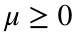we need to map out the acceptance region based on the likelihood ratio ordering principle.
The likelihood is. The log-likelihood is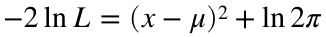. If we consider onlythen the likelihood is not defined for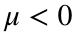. The log-likelihood is minimized, under the constraint, by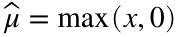. The minimized log-likelihood for the best-fit is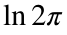for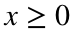and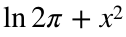for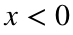. The log-likelihood ratio is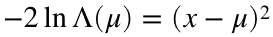forand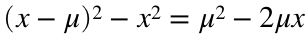for.
Below we see how the log-likelihood ratio looks for various small values of μ. For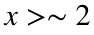the ratio is parabolic in an acceptance region of 90% or more, therefore the 90% CL F&C confidence intervals will coincide with the intervals defined by the log-likelihood contour defined by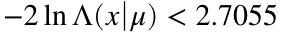, where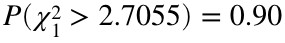. For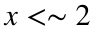the lower end of the acceptance region will be below 0, in the region where the log-likelihood is linear, and the computation of the interval is more complicated. We find numerically the threshold of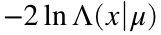, and the corresponding interval, that yields the specified acceptance (CL). For small enough x it won't be possible to define a 2-sided acceptance region, and there will only be an upper limit. This is the unifying feature of the F&C intervals - the automatic switch from 1-sided to 2-sided confidence intervals, always maintaining explicit coverage for all values of. Coverage means that regardles of the observed x, the derived interval will have a probability of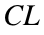of including the true value μ.
xm = linspace(-3,0,60);
xp = linspace(0,6,60);
figure
mu = 0;
plot(xm,mu^2-2*mu*xm,'k--','LineWidth',2), hold on
plot(xp,(xp-mu).^2,'k-')
mu = 0.5;
plot(xm,mu^2-2*mu*xm,'r--')
plot(xp,(xp-mu).^2,'r-')
mu = 1;
plot(xm,mu^2-2*mu*xm,'g--')
plot(xp,(xp-mu).^2,'g-')
mu = 2;
plot(xm,mu^2-2*mu*xm,'m--')
plot(xp,(xp-mu).^2,'m-')
xlabel('Observed mean $x$','Interpreter','latex')
ylabel('$-2\ln\Lambda(x|\mu)$','Interpreter','latex')
grid on
title('Log-likelihood ratio for $\mu\ge 0$','Interpreter','latex')
ca = gca;
ca.YLim = [0,9];
cs.Xlim = [-3,6];
yline(2.7055);
xline(0);
legend('$\mu=0, x<0$','$\mu=0, x\ge 0$','$\mu=0.5, x<0$','$\mu=0.5, x\ge 0$','$\mu=1, x<0$','$\mu=1, x\ge 0$','$\mu=2, x<0$','$\mu=2, x\ge 0$','Interpreter','latex')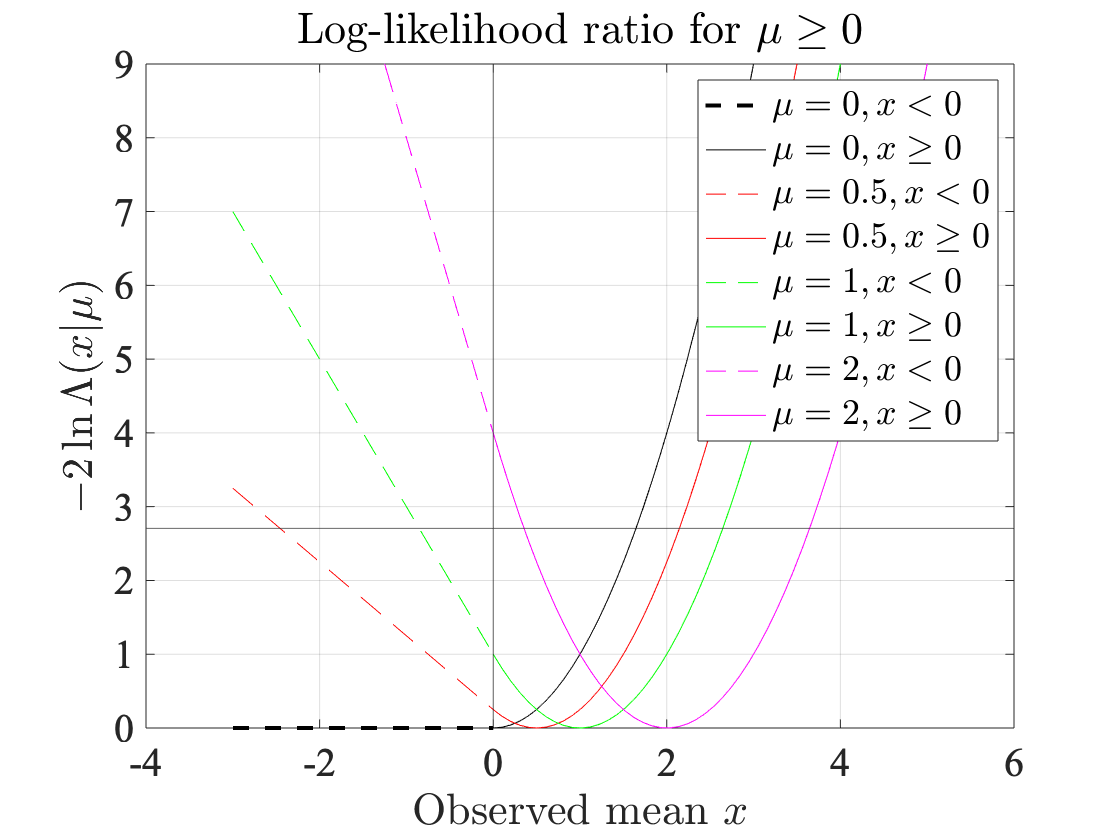## Form the confidence belt

Loop over true value μ for a given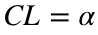. Check if the acceptance region α has both sides. If so, we can find the acceptance region by inverting the cdf of the normal distribution since the region is defined by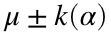. If the lower side has, the F&C prescription is to add regions of equal likelihood (or probability), i.e. we have to solve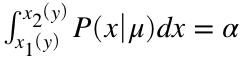for y, where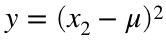forand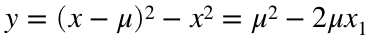for, in other words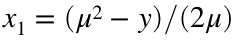and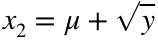.
mu = linspace(0,6,100);
mu(1) = 1E-6; % Avoid divide-by-zero problems with mu=0
alpha = 0.90
alpha = 0.9000
fun = @(y,mu,alpha) F(y,mu,alpha);
x1 = zeros(size(mu));
x2 = zeros(size(mu));
for i=1:length(mu)
p = normcdf(2*mu(i),mu(i))- normcdf(0,mu(i));
if p > alpha
% Lower side of acceptance region has x>=0
x1(i) = norminv((1-alpha)/2,mu(i));
x2(i) = norminv(1-(1-alpha)/2,mu(i));
else
% Lower side of acceptance region has x<0
fun = @(y,mu,alpha) F(y,mu,alpha);
y = fzero(@(y) fun(y,mu(i),alpha),5);
x1(i) = max(-5,(mu(i)^2-y)/(2*mu(i)));
x2(i) = mu(i) + sqrt(y);
ilast = i;
end
end

### Plot the confidence belt

The part of the belt that coincides with log-likelihood contours is shown in green. The part in black is affected by the constraint.
figure
plot(x1,mu,'k-'), hold on
plot(x2,mu,'k-')
plot(x1(ilast+1:end),mu(ilast+1:end),'g-')
plot(x2(ilast+1:end),mu(ilast+1:end),'g-')
ca = gca;
ca.XLim = [-2,4];
ca.YLim = [0,6];
xlabel('Measured mean $x$',"Interpreter","latex")
ylabel('Mean \mu')
grid on
title('Feldman and Cousins 90\% CL w/$$\mu\ge 0$$','Interpreter',"latex")

## Find limits directly

Find the upper limits as a function of the observed x without referring to tabulated confidence belts. Superimpose some points on the confidence belt to be sure it is done correctly.
xo = -2:0.1:4;
for i=1:length(xo)
muup(i) = find_mu_up(xo(i),alpha);
end
plot(xo,muup,'r+')
Find the lower limits as a function of the observed x without referring to tabulated confidence belts. Superimpose some points on the confidence belt to be sure it is done correctly.
for i=1:length(xo)
mudown(i) = find_mu_down(xo(i),alpha);
end
plot(xo,mudown,'ko')
Superimpose the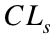95% and 90% CL upper limits
x = -2:0.1:4;
ul = iCLsUpperLimit(x,0.95);
plot(x,ul,'b-')
ul = iCLsUpperLimit(x,0.90);
plot(x,ul,'m-')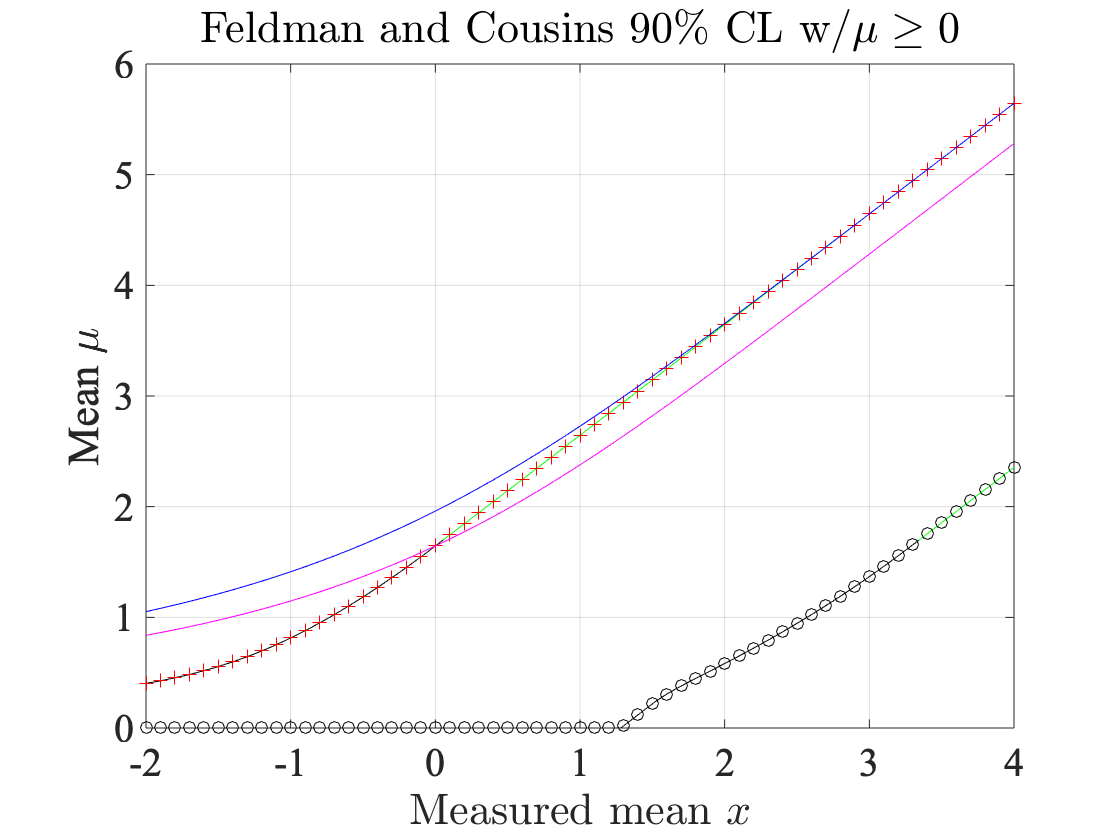legend('Belt left','Belt right','Belt left','Belt right','F&C UL','F&C LL','CLs 95% CL UL','CLs 90% CL UL','Location','best')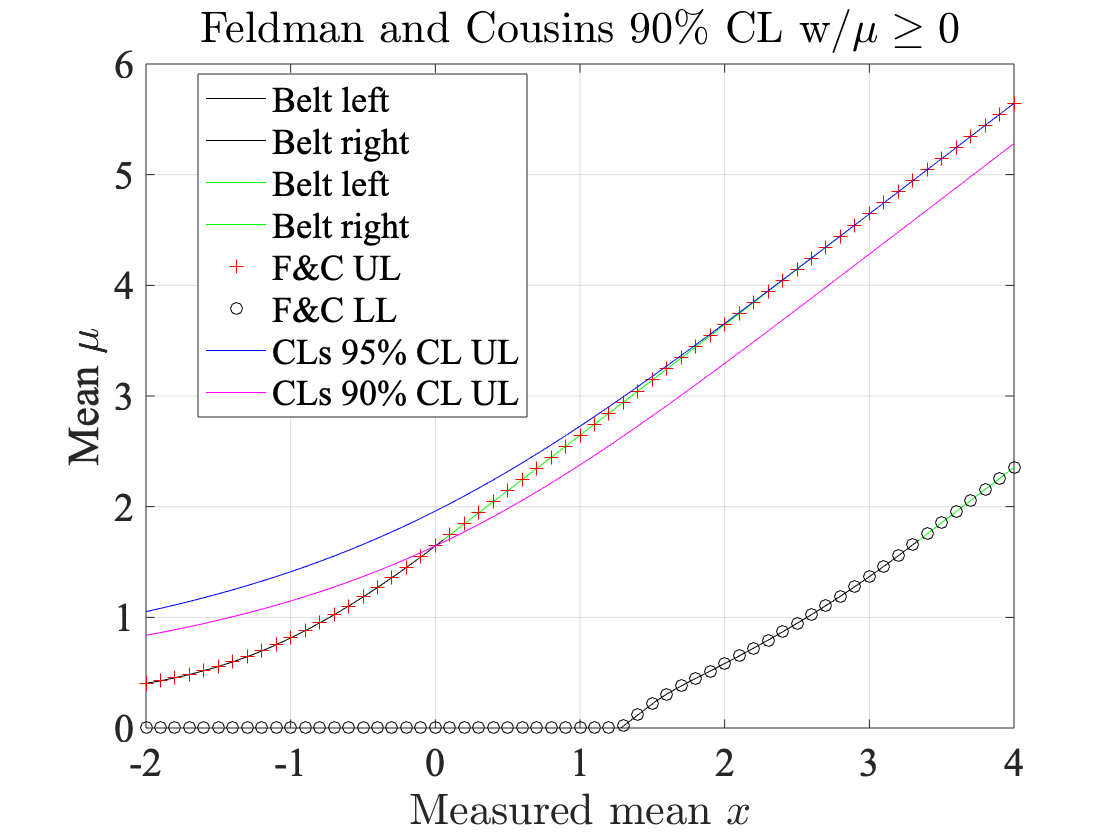## Reproduce Table X in the F&C article

Extend it to larger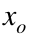and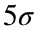CL
alphaTab=[0.6827,0.90,0.95,0.99,chi2cdf(5^2,1)];
fprintf('xo 68.27%% CL 90%% CL 95%% CL 99%% CL 5 sigma CL')
xo 68.27% CL 90% CL 95% CL 99% CL 5 sigma CL
for xo = -3:0.1:10
for i=1:length(alphaTab)
mudown(i) = find_mu_down(xo,alphaTab(i));
muup(i) = find_mu_up(xo,alphaTab(i));
end
fprintf('%4.1f %4.2f,%4.2f %4.2f,%4.2f %4.2f,%4.2f %4.2f,%4.2f %4.2f,%4.2f\n',...
xo,mudown(1),muup(1),mudown(2),muup(2),mudown(3),muup(3),mudown(4),muup(4),mudown(5),muup(5))
end
-3.0 0.00,0.04 0.00,0.26 0.00,0.42 0.00,0.80 0.00,2.72 -2.9 0.00,0.04 0.00,0.27 0.00,0.44 0.00,0.82 0.00,2.77 -2.8 0.00,0.04 0.00,0.28 0.00,0.45 0.00,0.84 0.00,2.82 -2.7 0.00,0.04 0.00,0.29 0.00,0.47 0.00,0.87 0.00,2.87 -2.6 0.00,0.05 0.00,0.30 0.00,0.48 0.00,0.89 0.00,2.92 -2.5 0.00,0.05 0.00,0.32 0.00,0.50 0.00,0.92 0.00,2.98 -2.4 0.00,0.05 0.00,0.33 0.00,0.52 0.00,0.95 0.00,3.03 -2.3 0.00,0.05 0.00,0.34 0.00,0.54 0.00,0.99 0.00,3.09 -2.2 0.00,0.06 0.00,0.36 0.00,0.56 0.00,1.02 0.00,3.15 -2.1 0.00,0.06 0.00,0.38 0.00,0.59 0.00,1.06 0.00,3.22 -2.0 0.00,0.07 0.00,0.40 0.00,0.62 0.00,1.10 0.00,3.28 -1.9 0.00,0.08 0.00,0.43 0.00,0.65 0.00,1.14 0.00,3.35 -1.8 0.00,0.09 0.00,0.45 0.00,0.68 0.00,1.19 0.00,3.42 -1.7 0.00,0.10 0.00,0.48 0.00,0.72 0.00,1.24 0.00,3.49 -1.6 0.00,0.11 0.00,0.52 0.00,0.76 0.00,1.29 0.00,3.57 -1.5 0.00,0.13 0.00,0.56 0.00,0.81 0.00,1.35 0.00,3.64 -1.4 0.00,0.15 0.00,0.60 0.00,0.86 0.00,1.41 0.00,3.72 -1.3 0.00,0.17 0.00,0.64 0.00,0.91 0.00,1.47 0.00,3.80 -1.2 0.00,0.20 0.00,0.70 0.00,0.97 0.00,1.54 0.00,3.88 -1.1 0.00,0.23 0.00,0.75 0.00,1.04 0.00,1.61 0.00,3.97 -1.0 0.00,0.27 0.00,0.81 0.00,1.10 0.00,1.68 0.00,4.06 -0.9 0.00,0.32 0.00,0.88 0.00,1.17 0.00,1.76 0.00,4.14 -0.8 0.00,0.37 0.00,0.95 0.00,1.25 0.00,1.84 0.00,4.23 -0.7 0.00,0.43 0.00,1.02 0.00,1.33 0.00,1.93 0.00,4.33 -0.6 0.00,0.49 0.00,1.10 0.00,1.41 0.00,2.01 0.00,4.42 -0.5 0.00,0.56 0.00,1.18 0.00,1.49 0.00,2.10 0.00,4.51 -0.4 0.00,0.64 0.00,1.27 0.00,1.58 0.00,2.19 0.00,4.61 -0.3 0.00,0.72 0.00,1.36 0.00,1.67 0.00,2.28 0.00,4.70 -0.2 0.00,0.81 0.00,1.45 0.00,1.77 0.00,2.38 0.00,4.80 -0.1 0.00,0.90 0.00,1.55 0.00,1.86 0.00,2.48 0.00,4.90 0.0 0.00,1.00 0.00,1.64 0.00,1.96 0.00,2.58 0.00,5.00 0.1 0.00,1.10 0.00,1.74 0.00,2.06 0.00,2.68 0.00,5.10 0.2 0.00,1.20 0.00,1.84 0.00,2.16 0.00,2.78 0.00,5.20 0.3 0.00,1.30 0.00,1.94 0.00,2.26 0.00,2.88 0.00,5.30 0.4 0.00,1.40 0.00,2.04 0.00,2.36 0.00,2.98 0.00,5.40 0.5 0.02,1.50 0.00,2.14 0.00,2.46 0.00,3.08 0.00,5.50 0.6 0.07,1.60 0.00,2.24 0.00,2.56 0.00,3.18 0.00,5.60 0.7 0.11,1.70 0.00,2.34 0.00,2.66 0.00,3.28 0.00,5.70 0.8 0.15,1.80 0.00,2.44 0.00,2.76 0.00,3.38 0.00,5.80 0.9 0.19,1.90 0.00,2.54 0.00,2.86 0.00,3.48 0.00,5.90 1.0 0.24,2.00 0.00,2.64 0.00,2.96 0.00,3.58 0.00,6.00 1.1 0.30,2.10 0.00,2.74 0.00,3.06 0.00,3.68 0.00,6.10 1.2 0.35,2.20 0.00,2.84 0.00,3.16 0.00,3.78 0.00,6.20 1.3 0.42,2.30 0.02,2.94 0.00,3.26 0.00,3.88 0.00,6.30 1.4 0.49,2.40 0.12,3.04 0.00,3.36 0.00,3.98 0.00,6.40 1.5 0.56,2.50 0.22,3.14 0.00,3.46 0.00,4.08 0.00,6.50 1.6 0.64,2.60 0.31,3.24 0.00,3.56 0.00,4.18 0.00,6.60 1.7 0.72,2.70 0.38,3.34 0.06,3.66 0.00,4.28 0.00,6.70 1.8 0.81,2.80 0.45,3.44 0.16,3.76 0.00,4.38 0.00,6.80 1.9 0.90,2.90 0.51,3.54 0.26,3.86 0.00,4.48 0.00,6.90 2.0 1.00,3.00 0.58,3.64 0.35,3.96 0.00,4.58 0.00,7.00 2.1 1.10,3.10 0.65,3.74 0.45,4.06 0.00,4.68 0.00,7.10 2.2 1.20,3.20 0.72,3.84 0.53,4.16 0.00,4.78 0.00,7.20 2.3 1.30,3.30 0.79,3.94 0.61,4.26 0.00,4.88 0.00,7.30 2.4 1.40,3.40 0.87,4.04 0.69,4.36 0.07,4.98 0.00,7.40 2.5 1.50,3.50 0.95,4.14 0.76,4.46 0.17,5.08 0.00,7.50 2.6 1.60,3.60 1.02,4.24 0.84,4.56 0.27,5.18 0.00,7.60 2.7 1.70,3.70 1.11,4.34 0.91,4.66 0.37,5.28 0.00,7.70 2.8 1.80,3.80 1.19,4.44 0.99,4.76 0.47,5.38 0.00,7.80 2.9 1.90,3.90 1.28,4.54 1.06,4.86 0.57,5.48 0.00,7.90 3.0 2.00,4.00 1.37,4.64 1.14,4.96 0.67,5.58 0.00,8.00 3.1 2.10,4.10 1.46,4.74 1.22,5.06 0.77,5.68 0.00,8.10 3.2 2.20,4.20 1.56,4.84 1.30,5.16 0.87,5.78 0.00,8.20 3.3 2.30,4.30 1.66,4.94 1.39,5.26 0.96,5.88 0.00,8.30 3.4 2.40,4.40 1.76,5.04 1.47,5.36 1.04,5.98 0.00,8.40 3.5 2.50,4.50 1.86,5.14 1.56,5.46 1.13,6.08 0.00,8.50 3.6 2.60,4.60 1.96,5.24 1.65,5.56 1.21,6.18 0.00,8.60 3.7 2.70,4.70 2.06,5.34 1.75,5.66 1.29,6.28 0.00,8.70 3.8 2.80,4.80 2.16,5.44 1.84,5.76 1.37,6.38 0.00,8.80 3.9 2.90,4.90 2.26,5.54 1.94,5.86 1.46,6.48 0.00,8.90 4.0 3.00,5.00 2.36,5.64 2.04,5.96 1.54,6.58 0.00,9.00 4.1 3.10,5.10 2.46,5.74 2.14,6.06 1.62,6.68 0.00,9.10 4.2 3.20,5.20 2.56,5.84 2.24,6.16 1.70,6.78 0.00,9.20 4.3 3.30,5.30 2.66,5.94 2.34,6.26 1.79,6.88 0.00,9.30 4.4 3.40,5.40 2.76,6.04 2.44,6.36 1.88,6.98 0.00,9.40 4.5 3.50,5.50 2.86,6.14 2.54,6.46 1.96,7.08 0.00,9.50 4.6 3.60,5.60 2.96,6.24 2.64,6.56 2.05,7.18 0.00,9.60 4.7 3.70,5.70 3.06,6.34 2.74,6.66 2.14,7.28 0.00,9.70 4.8 3.80,5.80 3.16,6.44 2.84,6.76 2.24,7.38 0.00,9.80 4.9 3.90,5.90 3.26,6.54 2.94,6.86 2.33,7.48 0.04,9.90 5.0 4.00,6.00 3.36,6.64 3.04,6.96 2.43,7.58 0.14,10.00 5.1 4.10,6.10 3.46,6.74 3.14,7.06 2.52,7.68 0.24,10.10 5.2 4.20,6.20 3.56,6.84 3.24,7.16 2.62,7.78 0.34,10.20 5.3 4.30,6.30 3.66,6.94 3.34,7.26 2.72,7.88 0.44,10.30 5.4 4.40,6.40 3.76,7.04 3.44,7.36 2.82,7.98 0.54,10.40 5.5 4.50,6.50 3.86,7.14 3.54,7.46 2.92,8.08 0.64,10.50 5.6 4.60,6.60 3.96,7.24 3.64,7.56 3.02,8.18 0.74,10.60 5.7 4.70,6.70 4.06,7.34 3.74,7.66 3.12,8.28 0.84,10.70 5.8 4.80,6.80 4.16,7.44 3.84,7.76 3.22,8.38 0.94,10.80 5.9 4.90,6.90 4.26,7.54 3.94,7.86 3.32,8.48 1.04,10.90 6.0 5.00,7.00 4.36,7.64 4.04,7.96 3.42,8.58 1.14,11.00 6.1 5.10,7.10 4.46,7.74 4.14,8.06 3.52,8.68 1.24,11.10 6.2 5.20,7.20 4.56,7.84 4.24,8.16 3.62,8.78 1.34,11.20 6.3 5.30,7.30 4.66,7.94 4.34,8.26 3.72,8.88 1.44,11.30 6.4 5.40,7.40 4.76,8.04 4.44,8.36 3.82,8.98 1.54,11.40 6.5 5.50,7.50 4.86,8.14 4.54,8.46 3.92,9.08 1.64,11.50 6.6 5.60,7.60 4.96,8.24 4.64,8.56 4.02,9.18 1.74,11.60 6.7 5.70,7.70 5.06,8.34 4.74,8.66 4.12,9.28 1.84,11.70 6.8 5.80,7.80 5.16,8.44 4.84,8.76 4.22,9.38 1.94,11.80 6.9 5.90,7.90 5.26,8.54 4.94,8.86 4.32,9.48 2.04,11.90 7.0 6.00,8.00 5.36,8.64 5.04,8.96 4.42,9.58 2.14,12.00 7.1 6.10,8.10 5.46,8.74 5.14,9.06 4.52,9.68 2.24,12.10 7.2 6.20,8.20 5.56,8.84 5.24,9.16 4.62,9.78 2.34,12.20 7.3 6.30,8.30 5.66,8.94 5.34,9.26 4.72,9.88 2.44,12.30 7.4 6.40,8.40 5.76,9.04 5.44,9.36 4.82,9.98 2.53,12.40 7.5 6.50,8.50 5.86,9.14 5.54,9.46 4.92,10.08 2.63,12.50 7.6 6.60,8.60 5.96,9.24 5.64,9.56 5.02,10.18 2.73,12.60 7.7 6.70,8.70 6.06,9.34 5.74,9.66 5.12,10.28 2.83,12.70 7.8 6.80,8.80 6.16,9.44 5.84,9.76 5.22,10.38 2.93,12.80 7.9 6.90,8.90 6.26,9.54 5.94,9.86 5.32,10.48 3.03,12.90 8.0 7.00,9.00 6.36,9.64 6.04,9.96 5.42,10.58 3.12,13.00 8.1 7.10,9.10 6.46,9.74 6.14,10.06 5.52,10.68 3.22,13.10 8.2 7.20,9.20 6.56,9.84 6.24,10.16 5.62,10.78 3.31,13.20 8.3 7.30,9.30 6.66,9.94 6.34,10.26 5.72,10.88 3.40,13.30 8.4 7.40,9.40 6.76,10.04 6.44,10.36 5.82,10.98 3.50,13.40 8.5 7.50,9.50 6.86,10.14 6.54,10.46 5.92,11.08 3.59,13.50 8.6 7.60,9.60 6.96,10.24 6.64,10.56 6.02,11.18 3.68,13.60 8.7 7.70,9.70 7.06,10.34 6.74,10.66 6.12,11.28 3.77,13.70 8.8 7.80,9.80 7.16,10.44 6.84,10.76 6.22,11.38 3.86,13.80 8.9 7.90,9.90 7.26,10.54 6.94,10.86 6.32,11.48 3.95,13.90 9.0 8.00,10.00 7.36,10.64 7.04,10.96 6.42,11.58 4.04,14.00 9.1 8.10,10.10 7.46,10.74 7.14,11.06 6.52,11.68 4.14,14.10 9.2 8.20,10.20 7.56,10.84 7.24,11.16 6.62,11.78 4.23,14.20 9.3 8.30,10.30 7.66,10.94 7.34,11.26 6.72,11.88 4.32,14.30 9.4 8.40,10.40 7.76,11.04 7.44,11.36 6.82,11.98 4.42,14.40 9.5 8.50,10.50 7.86,11.14 7.54,11.46 6.92,12.08 4.51,14.50 9.6 8.60,10.60 7.96,11.24 7.64,11.56 7.02,12.18 4.61,14.60 9.7 8.70,10.70 8.06,11.34 7.74,11.66 7.12,12.28 4.70,14.70 9.8 8.80,10.80 8.16,11.44 7.84,11.76 7.22,12.38 4.80,14.80 9.9 8.90,10.90 8.26,11.54 7.94,11.86 7.32,12.48 4.90,14.90 10.0 9.00,11.00 8.36,11.64 8.04,11.96 7.42,12.58 5.00,15.00

## Functions

Solving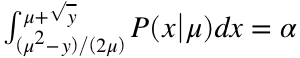for y allows us to find the confidence belt betweenandfor the case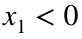. This could also be solved with a inline function.
function [value] = F(y,mu,alpha)
% Finding the zero of this function allows
% us to find x1(y) and x2(y) for a given alpha.
%
x1 = max(-5,(mu^2-y)/(2*mu));
x2 = mu + sqrt(y);
value = normcdf(x2,mu) - normcdf(x1,mu) - alpha;
end
Calculate the upper limit as directly as possible, i.e. without pre-tabulating the confidence belt results above. The upper limit of the confidence interval is given by the μ that places the observationat the left edge of the confidence belt. If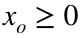then both ends of the confidence interval are within the parabolic part of the log-likelihood ratio and we can solve for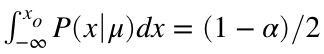. For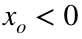we use that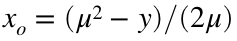andand solvefor μ. Make sure the interval that we search for the solution is more than wide enough to cover the solution! The upper limit will be in the neighborhood of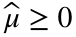plus the number of standard deviations corresponding to the confidence level (alpha).
function [mu] = find_mu_up(x1,alpha)
interval = [0,max(0,x1)+1.1*sqrt(chi2inv(alpha,1))];
if x1>=0
fun = @(mu,x1,alpha) normcdf(x1,mu)-(1-alpha)/2;
mu = fzero(@(mu) fun(mu,x1,alpha),interval);
else
fun2 = @(mu,x1,alpha) normcdf(mu+sqrt(mu^2-2*mu*x1),mu)-normcdf(x1,mu)-alpha;
mu = fzero(@(mu) fun2(mu,x1,alpha),interval);
end
end
Calculate the lower limit as directly as possible, i.e. without pre-tabulating the confidence belt results above. First of all, if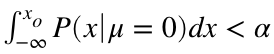, then we know the lower limit must be 0. Well above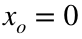, if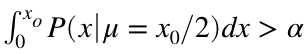, then we know that both ends of the confidence interval are within the parabolic part of the likelihood ratio and we can solve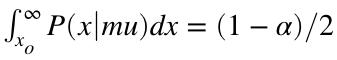for μ. In the remaining intermediate region we must solve forfor μ.
function [mu] = find_mu_down(x2,alpha)
p = normcdf(x2,0);
if p<alpha
mu = 0;
else
interval = [0,max(1,x2)];
p = normcdf(x2,x2/2)-normcdf(0,x2/2);
if p>=alpha
fun = @(mu,x2,alpha) normcdf(x2,mu,'upper')-(1-alpha)/2;
mu = fzero(@(mu) fun(mu,x2,alpha),interval);
else
fun = @(mu,x2,alpha) normcdf(x2,mu)-normcdf((2*x2*mu-x2^2)/(2*mu),mu)-alpha;
mu = fzero(@(mu) fun(mu,x2,alpha),interval);
end
end
end
Calculate theupper limit for the unit Gaussian pdf (use this internal copy to make the live script self-contained).
function [uplim,pmu,ompb] = iCLsUpperLimit(x,CL)
%iCLsUpperLimit Compute CLs upper limit.
% [uplim,pmu,ompb] = CLsUpperLimit(x,CL) finds the upper limit
% at the CL confidence level using CLs=pmu/(1-pb) for the unit
% gaussian measurement x with the null (background-only) hypothesis
% at 0 and requiring the signal to be positive. The p-value for the
% signal plus background hypothesis, pmu, and ompb, the p-value for the
% null hypothesis, often called 1-p_b, are also returned.
%
% If x is a list of observations, lists of upper limits and
% p-values are returned.
ompb = normcdf(x,0);
for i=1:length(x)
uplim(i) = fzero(@(mu) normcdf(x(i),mu)/ompb(i)-(1-CL),x(i));
pmu(i) = normcdf(x(i),uplim(i));
end
end